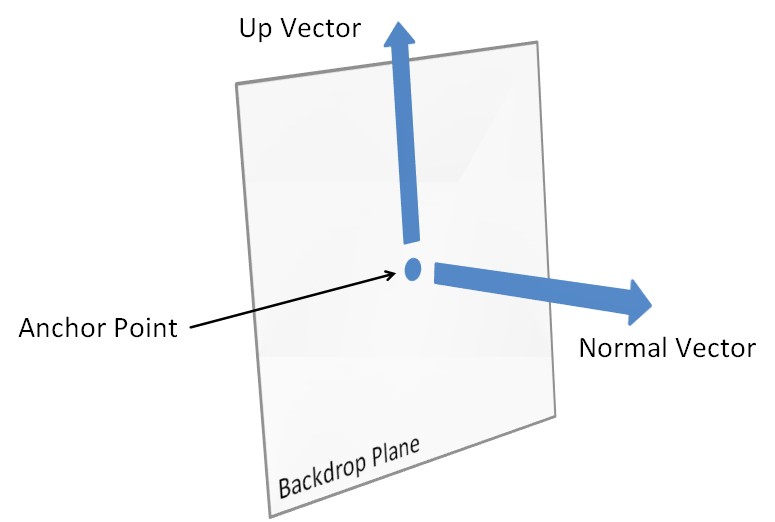# `<up>` (Up Vector)

This element defines a vector representing up. To be more precise, this attribute defines a vector representing up in relation to the face of the backdrop plane.

example:Parent Elements

`<backdrop>`5.1.7.2)

Attributes

Description

`<dx>` (Distance along X-axis in 3D)

Distance along X-axis in 3D

The possible values for this attribute are defined by the ST_Coordinate simple type (§5.1.12.16).

`<dy>` (Distance along Y-axis in 3D)

Distance along Y-axis in 3D

The possible values for this attribute are defined by the ST_Coordinate simple type (§5.1.12.16).

`<dz>` (Distance along Z-axis in 3D)

Distance along Z-axis in 3D

The possible values for this attribute are defined by the ST_Coordinate simple type (§5.1.12.16).

The following XML Schema fragment defines the contents of this element:

``````<complexType name="CT_Vector3D">
<attribute name="dx" type="ST_Coordinate" use="required"/>
<attribute name="dy" type="ST_Coordinate" use="required"/>
<attribute name="dz" type="ST_Coordinate" use="required"/>
</complexType>``````